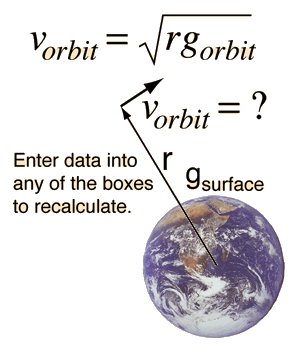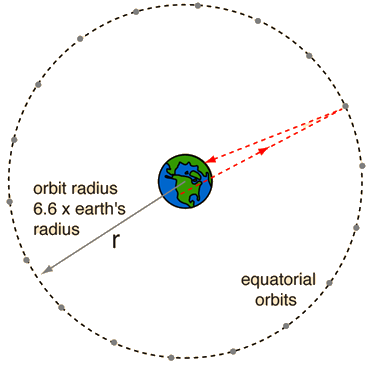# Earth Orbit Velocity

The velocity of a satellite in circular orbit around the Earth depends upon the radius of the orbit and the acceleration of gravity at the orbit.Above the earth's surface at a height of h =m = x 106 m, which corresponds to a radius r = x earth radius, the acceleration of gravity is g = m/s2 = x g on the earth's surface. At the specified orbit radius, the required orbit velocity is v =m/s=km/h=mi/h. The period of the orbit is minutes = hours.
Index

Gravity concepts

 HyperPhysics***** Mechanics ***** Rotation R Nave
Go Back

# Syncom Satellites

Communication satellites are most valuable when they stay above the same point on the earth, in what are called "geostationary orbits". This occurs when the orbital period is 24 hours. From an orbit velocity calculation, it can be seen that a satellite at a radius 6.62 times the Earth's radius will have a period of 24 hours. These satellites are some 35,900 kilometers or about 22,300 miles above the Earth's surface.The minimum transit time to a syncom satellite and back from the surface of the Earth is about a quarter of a second. Any kind of electromagnetic wave travels at the speed of light. So in satellite-linked communication, there will be a minimum of a quarter of a second delay.
Index

Gravity concepts

 HyperPhysics***** Mechanics ***** Rotation R Nave
Go Back